# Consideration of Channel Distortion and Equalization

## Ideal channel equalizer

For a transmission system whose channel frequency response  $H_{\rm K}(f)$  causes severe distortion,  we assume the following block diagram  (upper graph)  and  equivalent circuit  (lower graph).  To these representations the following is to be noted: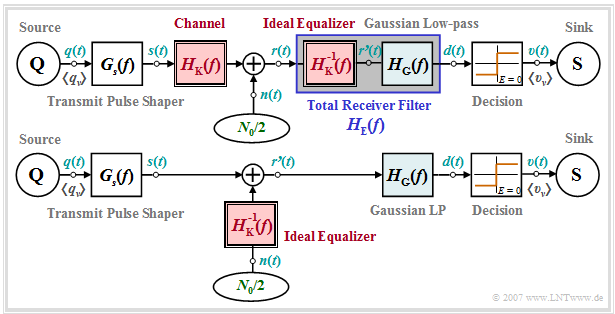Block diagram & equivalent circuit diagram for consideration of a channel frequency response
• The receiver filter  $H_{\rm E}(f)$  is – at least mentally – composed of an  ideal equalizer  $1/H_{\rm K}(f)$  and a low-pass filter  $H_{\rm G}(f)$.  For the latter we use in this chapter exemplarily a  Gaussian low-pass  with the cutoff frequency  $f_{\rm G}$.
• If we now move the ideal equalizer – again purely mentally – to the left side of the noise addition point,  nothing changes with respect to the S/N ratio before the decision device and with respect to the error probability compared to the block diagram drawn above.
• From the equivalent diagram below it can be seen that the channel frequency response  $H_{\rm K}(f)$  does not change anything with respect to the signal component  $d_{\rm S}(t)$  of the detection signal if it is fully compensated with  $1/H_{\rm K}(f)$.
• The degradation due to the frequency response  $H_{\rm K}(f)$  is rather shown by a significant increase of the detection noise power, i.e. the variance of the noise component  $d_{\rm N}(t)$  – originating from the noise signal  $n(t)$  at the receiver input:
$$\sigma_d^2 = \frac{N_0}{2} \cdot \int_{-\infty}^{+\infty} |H_{\rm E}(f)|^2 \,{\rm d} f = \frac{N_0}{2} \cdot \int_{-\infty}^{+\infty} \frac{1}{|H_{\rm K}(f)|^2}\cdot |H_{\rm G}(f)|^2 \,{\rm d} f \hspace{0.05cm}.$$
• The prerequisite for a finite noise power  $\sigma_d^2$  is that the Gaussian low-pass  $H_{\rm G}(f)$  attenuates the noise  $n(t)$  at  (very)  high frequencies more than it is raised by the ideal equalizer  $1/H_{\rm K}(f)$.

Note:   The channel frequency response  $H_{\rm K}(f)$  must be equalized for magnitude and phase,  but only in a limited frequency range specified by  $H_{\rm G}(f)$ .  However,  a complete phase equalization is only possible at the expense of a  (frequency-independent)  delay time,  which will not be considered further in the following.

$\text{Example 1:}$  We consider again a binary system with NRZ rectangular pulses and a Gaussian receiver filter  $H_{\rm E}(f) = H_{\rm G}(f)$  with  (normalized)  cutoff frequency  $f_\text{G, opt} \cdot T = 0.4$.  Due to this unfavorable receiver filter  $H_{\rm E}(f)$  intersymbol interference  $\rm (ISI)$ occurs in all variants presented here.

• The middle graph shows the eye diagram of the signal component  $d_{\rm S}(t)$ of the detection signal for this case – i.e. without taking the noise into account.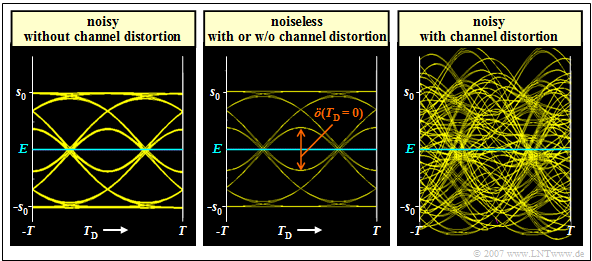Binary eye diagrams  (noisy or noiseless)  with intersymbol interferences

⇒   The left  (noisy)  diagram is obtained for the ideal channel, i.e., for

$$H_{\rm K}(f) = 1 \ \ ⇒ \ \ 1/H_{\rm K}(f) = 1.$$

It takes into account the AWGN noise,  but here it was assumed to be very small,  $10 \cdot {\rm lg}\hspace{0.1cm} E_{\rm B}/N_0 = 30 \ \rm dB$.  For this configuration,  the  worst-case  SNR it was determined by simulation:

$$10 \cdot {\rm lg}\hspace{0.1cm}\rho_{\rm U}\approx 26.8\,{\rm dB} \hspace{0.3cm}\Rightarrow \hspace{0.3cm} p_{\rm U}< 10^{-40}\hspace{0.05cm}.$$

⇒   The right  (also noisy)  diagram applies to a  coaxial cable,  where the characteristic cable attenuation  $a_\star = 40 \ \rm dB$.  This results in significantly worse system performance for the same  $E_{\rm B}/N_0$:

$$10 \cdot {\rm lg}\hspace{0.1cm}\rho_{\rm U}\approx -4.6\,{\rm dB} \hspace{0.3cm}\Rightarrow \hspace{0.3cm} p_{\rm U}\approx 0.28\hspace{0.05cm}.$$

This result can be interpreted as follows:

• Assuming an ideal channel equalizer  $1/H_{\rm K}(f)$,  the same  "eye diagram without noise"  (left diagram)  results for the distorting channel as for the ideal, distortion-free channel  $H_{\rm K}(f) = 1$  (middle graph).
• Channel equalization  $1/H_{\rm K}(f)$  extremely amplifies the noise component  $d_{\rm N}(t)$  of the detection signal.  In the right-hand example,  equally strong equalization is required over a wide frequency range because of the strong distortion.
• The noise power  $\sigma_d^2$  here is larger by a factor of  $1300$  than in the left constellation  $($no distortion   ⇒   no equalization$)$.  Thus,  the error probability results in  $p_{\rm S}\approx p_{\rm U}\approx 50 \%$.
• An acceptable worst-case error probability results only with smaller noise power density  $N_0$.  For example,  with  $10 \cdot {\rm lg}\hspace{0.1cm} E_{\rm B}/N_0 = 50 \ \rm dB$  $($instead of $30 \ \rm dB)$  the following result is obtained:
$$10 \cdot {\rm lg}\hspace{0.1cm}\rho_{\rm U} = -4.6 +20 \approx 15.4\,{\rm dB} \hspace{0.3cm}\Rightarrow \hspace{0.3cm} p_{\rm U}\approx 2 \cdot 10^{-9} \hspace{0.3cm}\Rightarrow \hspace{0.3cm} p_{\rm S} \ge p_{\rm U}/4 \approx 0.5 \cdot 10^{-9}\hspace{0.05cm}.$$

## Increase of the noise power by linear equalization

The eye diagrams on the last section impressively document the increase of the noise power  $\sigma_d^2$  with unchanged vertical eye opening,  if one compensates the channel frequency response  $H_{\rm K}(f)$  on the receiving side by its inverse.  This result shall now be interpreted in terms of the noise power density  ${\it \Phi}_{d{\rm N}}(f)$  after the receiver filter  (before the decision),  with the following settings:

• Let the channel be a  coaxial cable  with the magnitude frequency response
$$|H_{\rm K}(f)| = {\rm exp}\left [- a_{\star}\cdot \sqrt{2 f T}\hspace{0.05cm} \right ]\hspace{0.2cm}{\rm with}\hspace{0.2cm} a_{\star} = 1.7\,\,{\rm Np}\hspace{0.2cm} ({\rm corresponding \hspace{0.2cm} to} \hspace{0.2cm} 15\,\,{\rm dB}) \hspace{0.05cm}.$$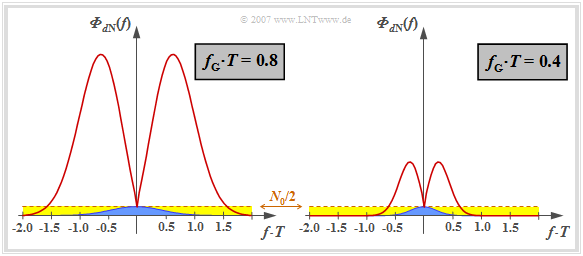Noise power-spectral density before the decision due to distorting channel.
Note that here,  for reasons of presentation,  the characteristic cable attenuation of  $a_\star = 15 \ \rm dB$   $($corresponding to  $1.7 \ \rm Np)$  is chosen to be significantly smaller than in the right eye diagram in  Example 1  in the last section  $($valid for  $a_\star = 40 \ \rm dB)$.
• The  ideal channel equalizer  $1/H_{\rm K}(f)$  compensates the channel frequency response completely.  No statement is made here about the realization of the attenuation and phase equalization.
$$|H_{\rm G}(f)| = {\rm exp}\left [- \pi \cdot \left (\frac{f }{2 f_{\rm G}}\right )^2 \right ] \hspace{0.05cm}.$$

Thus,  the noise power-spectral density before the decision is:

$${\it \Phi}_{d{\rm N}}(f) = \frac{N_0}{2} \cdot \frac{|H_{\rm G }(f)|^2}{|H_{\rm K}(f)|^2}$$
$$\Rightarrow \hspace{0.3cm} {\it \Phi}_{d{\rm N}}(f) = \frac{N_0}{2} \cdot {\rm exp}\left [2 \cdot a_{\star}\cdot \sqrt{2 f T} - {\pi}/{2} \cdot \left ({f }/{f_{\rm G}}\right )^2 \right ] \hspace{0.05cm}.$$

This curve is shown for two  (normalized)  cutoff frequencies

• $f_\text{G} \cdot T = 0.8$  (on the left),
• $f_\text{G} \cdot T = 0.4$  (on the right).

Let us first consider the left graph for the (normalized) cutoff frequency  $f_\text{G} \cdot T = 0.8$,  which  – according to the calculations in the  last chapter  –  represents the optimum for the ideal channel   ⇒   $H_{\rm K}(f) = 1$.

1. The constant noise power density  $N_0/2$  at the receiver input is highlighted in yellow.  With an ideal channel,  this is limited by the Gaussian receiver filter  $H_{\rm E}(f) = H_{\rm G}(f)$  and results in the detection noise power  $\sigma_d^2$  (indicated by the blue area in the graph).

2. If – as usual for  "Wireline Transmission Technology"  – higher frequencies are strongly attenuated,  $|H_{\rm E}(f)| = |H_{\rm G}(f)|/|H_{\rm K}(f)|$  increases very strongly due to the ideal channel equalizer before the attenuating influence of the Gaussian filter becomes effective for  $f \cdot T \ge 0.6$  $($only valid for  $a_\star = 15 \ \rm dB$  and  $f_\text{G} \cdot T = 0.8)$.

3. The noise power  $\sigma_d^2$  is now equal to the area under the red curve,  which is about a factor of  $28$  larger than the blue area.  The effects of these different noise powers can be seen qualitatively in the left and right eye diagrams on the last section,  but for  $a_\star = 40 \ \rm dB$.

The right graph shows the noise power density  ${\it \Phi}_{d{\rm N}}(f)$  for the normalized cutoff frequency  $f_\text{G} \cdot T = 0.4$. Here the noise power is only increased by a factor of  $9$  by the ideal channel equalizer  (ratio between the area under the red curve and the blue area).

$\text{Conclusion:}$  From the above graph and the previous explanations it is already clear that with distorting channel   ⇒   $H_{\rm K}(f) \ne 1$ the cutoff frequency  $f_\text{G} \cdot T = 0.8$  of the Gaussian low-pass filter $H_{\rm G}(f)$ will no longer be optimal after the ideal channel equalizer  $1/H_{\rm K}(f)$.

## Optimization of the cutoff frequency

The graph shows the signal-to-noise ratios as a function of the cutoff frequency  $f_{\rm G}$  of the overall Gaussian frequency response  $H_{\rm G}(f) = H_{\rm K}(f) \cdot H_{\rm E}(f)$.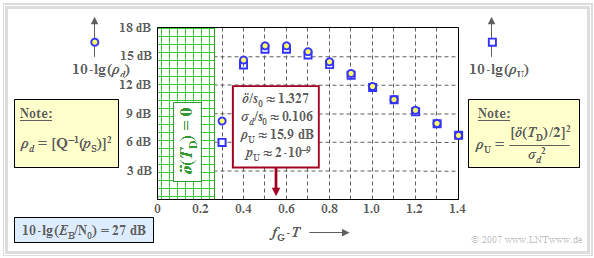Optimal cutoff frequency of the Gaussian low-pass with distorting channel  $(a_\star = 15 \ \rm dB).$
⇒   The circles show the dB values for  $10 \cdot {\rm lg}\hspace{0.1cm} \rho_d$   ⇒   "mean detection SNR"   ⇒   measure of mean error probability  $p_{\rm S}$.
⇒   The squares show the dB values for  $10 \cdot {\rm lg}\hspace{0.1cm} \rho_{\rm U}$   ⇒   "worst-case SNR"   ⇒   measure of worst-case error probability  $p_{\rm U}$.

This graphic is valid for

• AWGN noise with  $10 \cdot {\rm lg}\hspace{0.1cm} E_{\rm B}/N_0 = 27 \ \rm dB$,  with  $E_{\rm B} = s_0^2 \cdot T$   ⇒   NRZ rectangular pulses.

One can see by comparison with the  corresponding plot  in the last chapter, which was valid for  $H_{\rm K}(f) = 1$,  $10 \cdot {\rm lg}\hspace{0.1cm} E_{\rm B}/N_0 = 13 \ \rm dB$:

• Even with a highly distorting channel,  $\rho_{\rm U}$  is a suitable lower bound for  $\rho_d$   ⇒   $\rho_{d} \ge \rho_{\rm U}$. Correspondingly,  $p_{\rm U} \ge p_{\rm S}$  is also a reasonable upper bound for  $p_{\rm S}$.
• For the considered cable attenuation  $a_\star = 15 \ \rm dB$,  the cutoff frequency  $f_\text{G} \cdot T \approx 0.55$  is optimal and  $\ddot{o}/s_0 \approx 1.327$  and  $\sigma_d/s_0 \approx 0.106$  hold.
• This gives the  worst-case SNR  $10 \cdot {\rm lg}\hspace{0.1cm} \rho_{\rm U} \approx \ \rm 15.9 \ dB$  and the worst–case error probability  $p_{\rm U} \approx 2 \cdot 10^{-9}.$
• A smaller cutoff frequency would result in a significantly smaller eye opening without equally reducing  $\sigma_d$.  For  $f_\text{G} \cdot T = 0.4$:
$$\ddot{o}/s_0 \approx 0.735,\hspace{0.2cm}\sigma_d/s_0 \approx 0.072$$
$$\hspace{0.3cm}\Rightarrow \hspace{0.3cm} 10 \cdot {\rm lg}\hspace{0.1cm}\rho_{\rm U}\approx 14.1\,{\rm dB} \hspace{0.3cm}\Rightarrow \hspace{0.3cm} p_{\rm U}\approx 1.8 \cdot 10^{-7}\hspace{0.05cm}.$$
• If the cutoff frequency  $f_\text{G}$  is too large, the noise is limited less effectively.
For example,  the values for the cutoff frequency are  $f_\text{G} \cdot T =0.8$:
$$\ddot{o}/s_0 \approx 1.819,\hspace{0.2cm}\sigma_d/s_0 \approx 0.178\hspace{0.3cm}\Rightarrow \hspace{0.3cm} 10 \cdot {\rm lg}\hspace{0.1cm}\rho_{\rm U}\approx 14.2\,{\rm dB} \hspace{0.3cm}\Rightarrow \hspace{0.3cm} p_{\rm U}\approx 1.7 \cdot 10^{-7}\hspace{0.05cm}.$$
• The optimal values  $10 \cdot {\rm lg}\hspace{0.1cm} \rho_{d} \approx 16.2 \ \rm dB$  and  $10 \cdot {\rm lg}\hspace{0.1cm} \rho_{\rm U} \approx \ \rm 15.9 dB$  are much more pronounced than for the ideal channel.

When comparing the signal-to-noise ratios,  however,  it must be taken into account that  $10 \cdot {\rm lg}\hspace{0.1cm} E_{\rm B}/N_0 = 27 \ \rm dB$  is the basis here;  in contrast,  $10 \cdot {\rm lg}\hspace{0.1cm} E_{\rm B}/N_0 = 13 \ \rm dB$  was assumed in the  "corresponding graph"  for the ideal channel.

## Optimal cutoff frequency as a function of cable attenuation

We further consider

• a binary system with NRZ transmission pulses   ⇒   $E_{\rm B} = s_0^2 \cdot T$,
• a coaxial cable $H_{\rm K}(f)$,  characteristic attenuation  $a_\star$,
• a Gaussian total frequency response  $H_{\rm G}(f) = H_{\rm K}(f) \cdot H_{\rm E}(f)$.

The blue circles  (left axis labels)  mark the optimal cutoff frequencies  $f_\text{G, opt}$  for the respective cable attenuation  $a_\star$.

In addition,  the graph shows with red squares the  "system efficiency"  (at peak limitation)   $\eta$,  which is the ratio of the SNR  $\rho_{d}$  achievable with the considered configuration to the maximum possible S/N ratio  $\rho_{d, \ {\rm max}}$.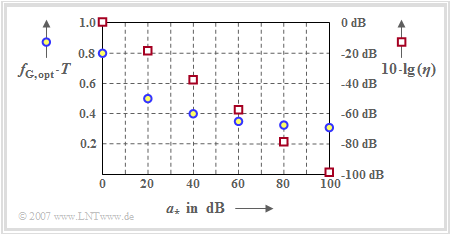Optimal cutoff frequency and system efficiency as a function of characteristic cable attenuation. In particular:
⇒   $10 · \lg \eta\hspace{0.05cm}(a_\star = 0 \ \rm dB) = -1.4 \ dB$;
⇒   $10 · \lg \eta\hspace{0.05cm}(a_\star = 80 \ \rm dB) = -78.2 \ dB.$

Replacing  $\rho_d$  by  $\rho_{\rm U}$, i.e.,  $p_{\rm S}$  by  $p_{\rm U}$, the system efficiency can be represented as follows:

$$\eta = \eta_{\rm A}=\frac{\rho_d}{\rho_{d, \hspace{0.05cm}{\rm max \hspace{0.05cm}|\hspace{0.05cm} A}}}= \frac{\rho_d}{2 \cdot E_{\rm B}/N_0}\approx \frac{\rho_{\rm U}}{2 \cdot E_{\rm B}/N_0}.$$

One can see from the arrangement of the blue circles:

• The optimal cutoff frequency  $f_\text{G, opt}$  depends significantly on the strength of the coaxial cable distortions,    exclusively on the characteristic cable attenuation  $a_\star$  at half the bit rate.
• The larger the cable attenuation  $a_\star$  and thus the noise influence,  the lower the optimal cutoff frequency  $f_\text{G, opt}$.
• However,  always  $f_\text{G, opt} > 0.27/T$.  Otherwise,  the eye would be closed,  equivalent to the  "worst–case error probability"  $p_{\rm U} = 0.5$.

Let us now discuss the dependence of the  system efficiency  $\eta$  (red squares)  on the characteristic cable attenuation  $a_\star$.  The right ordinate starts at the top at  $0 \ \rm dB$  and extends downward to  $-100 \ \rm dB$.

As will now be illustrated by some numerical examples,  the representation  $\eta = \eta\hspace{0.05cm}(a_\star)$ avoids some problems arising from the wide value range of S/N ratios:

• The ordinate value  $10 \cdot {\rm lg}\hspace{0.1cm} \eta\hspace{0.05cm}(a_\star = 0 \ \rm dB) = -1.4 \ \rm dB$  states that the best possible Gaussian low-pass with cutoff frequency  $f_\text{G} \cdot T = 0.8$  at ideal channel is  $1.4 \ \rm dB$  worse than the optimal  (matched filter)  receiver.
• If we assume an ideal channel  $(a_\star = 0 \ \rm dB)$  and  $10 \cdot {\rm lg}\hspace{0.1cm} E_{\rm B}/N_0 = 10 \ \rm dB$,  the above equation also states that this configuration will lead to the following  "worst-case error probability":
$$10 \cdot {\rm lg}\hspace{0.1cm}\rho_{\rm U} = 10 \cdot {\rm lg}\hspace{0.1cm}{E_{\rm B}}/{N_0} + 10 \cdot {\rm lg}\hspace{0.1cm}(2) + 10 \cdot {\rm lg}\hspace{0.1cm}(\eta) \approx 10\,{\rm dB} \hspace{0.1cm}+\hspace{0.1cm}3\,{\rm dB} \hspace{0.1cm}-\hspace{0.1cm}1.4\, {\rm dB}= 11.6\,{\rm dB} \hspace{0.3cm}\Rightarrow \hspace{0.3cm} p_{\rm U}\approx 7 \cdot 10^{-5}\hspace{0.05cm}.$$
• Accordingly, if this worst-case error probability  $p_{\rm U} = 7 \cdot 10^{-5}$   ⇒   $10 \cdot {\rm lg}\hspace{0.1cm} \rho_{\rm U} = 11.6 \ \rm dB$  is not to be exceeded for the channel with characteristic cable attenuation  $a_\star = 80 \ \rm dB$,  then the following must apply to the  $E_{\rm B}/N_0$  ratio:
$10 \cdot {\rm lg}\hspace{0.1cm}{E_{\rm B}}/{N_0} \ge 11.6\,{\rm dB} \hspace{0.1cm}-3\,{\rm dB} \hspace{0.1cm}-\hspace{0.1cm}(-78.2)\,{\rm dB}= 86.8\,{\rm dB} \hspace{0.2cm} \Rightarrow \hspace{0.2cm}{E_{\rm B}}/{N_0}\approx 5 \cdot 10^{8}\hspace{0.05cm}.$
• To achieve this,  however,  the cutoff frequency of the Gaussian low-pass filter must be lowered to  $f_{\rm G}= 0.33/T$  according to the blue circles in the graph.